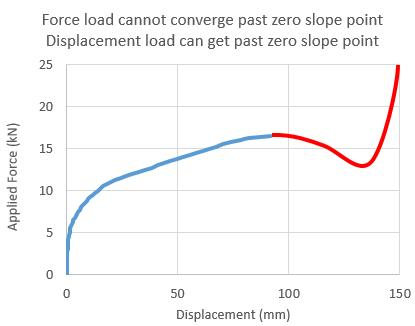peteroznewman
Subscriber

Hi Mekefime,

Here is the Force-Displacement plot for the above run in blue.I drew in the red curve by hand to illustrate what a displacement load can do that a force load cannot.
The red curve is not data, it's just a sketch. I am running the model with an actual displacement load now.

The force load was asked to find an equilibrium at each incremental increase in load from zero to 25 kN. The problem is there is no nearby equilibrium for a small increase in force past 16.5 kN.  You have to wait till you crush the 154 mm tall tube down to nothing and the vertical tube starts pushing on the bottom of the horizontal tube.

A displacement load has no problem following the solution past the point of zero slope on the force-displacement curve, because there is an equilibrium past that point, it just has less force that the earlier points.

If you request the reaction force from the displacement boundary condition that is set to Y = -150 mm, you can plot the force-displacement curve.

Regards,

Peter